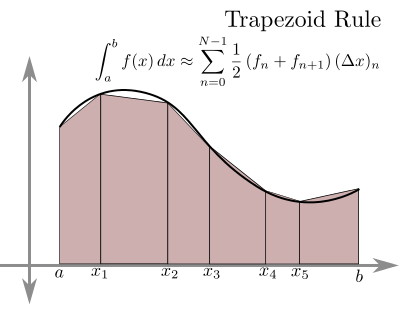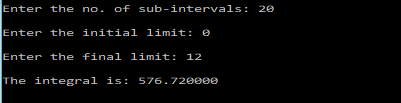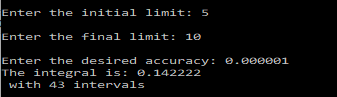# Trapezoidal Rule – C Program

Trapezoidal Rule is a Numerical technique to find the definite integral of a function.The function is divided into many sub-intervals and each interval is approximated by a Trapezium. Then the area of trapeziums is calculated to find the integral which is basically the area under the curve. THe more is the number of trapeziums used, the better is the approximation.

### Formula:$\int_a^bf(x)dx\approx. \frac{h}{2}(f(a)+2f(a+h)+2f(a+2h)+....+f(b))$

The following is a simple C Program that uses the trapezoidal rule to find the definite integral of a function.
Users, will have to change the function f in the following program to the function whose integral they want to find.

### PROGRAM (SIMPLE VERSION):

/*********************************
********TRAPEZOIDAL RULE*********
2017 (c) Manas Sharma - https://bragitoff.com
********************************/
#include<stdio.h>
#include<math.h>

/* Define the function to be integrated here: */
double f(double x){
return x*x;
}

/*Program begins*/
main(){
int n,i;
double a,b,h,x,sum=0,integral;
/*Ask the user for necessary input */
printf("\nEnter the no. of sub-intervals: ");
scanf("%d",&n);
printf("\nEnter the initial limit: ");
scanf("%lf",&a);
printf("\nEnter the final limit: ");
scanf("%lf",&b);
/*Begin Trapezoidal Method: */
h=fabs(b-a)/n;
for(i=1;i<n;i++){
x=a+i*h;
sum=sum+f(x);
}
integral=(h/2)*(f(a)+f(b)+2*sum);
printf("\nThe integral is: %lf\n",integral);
}



### OUTPUT:The above program returns a better approximation to the interval as the number of sub-intervals is increased. This might work for some applications, however, sometimes one might not want to deal with the number of sub-intervals, but rather the accuracy upto a certain decimal places. What I mean by accuracy is that sometimes you might just want the approximate value of integral upto a few decimal place. So you will have to keep on increasing the number of sub-intervals and check the value of the integral. If the integral for two subsequent no. of sub-intervals is within the accuracy/tolerance limit given by the user(or set by you) then the integral should be printed out.

The following program illustrates the process of achieving what I just explained and also uses a function called ‘trapezoidal’ that handles the integration part.

### PROGRAM (Better Version):

/******************************************
****TRAPEZOIDAL RULE USING FUNCTION*******
2017 (c) Manas Sharma - https://bragitoff.com
*****************************************/
#include<stdio.h>
#include<math.h>

/* Define the function to be integrated here: */
double f(double x){
return x*x;
}

/*Function definition to perform integration by Trapezoidal Rule */
double trapezoidal(double f(double x), double a, double b, int n){
double x,h,sum=0,integral;
int i;
h=fabs(b-a)/n;
for(i=1;i<n;i++){
x=a+i*h;
sum=sum+f(x);
}
integral=(h/2)*(f(a)+f(b)+2*sum);
return integral;
}

/*Program begins*/
main(){
int n,i=2;
double a,b,h,eps,sum=0,integral,integral_new;

/*Ask the user for necessary input */
printf("\nEnter the initial limit: ");
scanf("%lf",&a);
printf("\nEnter the final limit: ");
scanf("%lf",&b);
printf("\nEnter the desired accuracy: ");
scanf("%lf",&eps);
integral_new=trapezoidal(f,a,b,i);

/* Perform integration by trapezoidal rule for different number of sub-intervals until they converge to the given accuracy:*/
do{
integral=integral_new;
i++;
integral_new=trapezoidal(f,a,b,i);
}while(fabs(integral_new-integral)>=eps);

printf("The integral is: %lf\n with %d intervals",integral_new,i);
}



### OUPTUT:[wpedon id="7041" align="center"]

## One thought on “Trapezoidal Rule – C Program”

1. Can I run this code on Matlab too?